Courses

# Assignment - Triangles, Class 9 Mathematics Class 9 Notes | EduRev

Created by: Learners Habitat

## Class 9 : Assignment - Triangles, Class 9 Mathematics Class 9 Notes | EduRev

The document Assignment - Triangles, Class 9 Mathematics Class 9 Notes | EduRev is a part of Class 9 category.
All you need of Class 9 at this link: Class 9

FILL IN THE BLANKS :

(a) Sides opposite to the equal angles of a triangle are______.
(b) Angles opposite to the equal sides of a triangle are______.
(c) In an isosceles triangle ABC with AB = AC, if BD and CE are its altitudes, then BD is ______CE.
(d) If altitudes CE and BF of a triangle ABC are equal, then AB =______.
(e) In right triangles PQR and DEF, if hypotenuse PQ = hypotenuse EF and side PR = DE, then ΔPQR  Δ______.
(f) In a triangle ABC, if BC = AB and ∠C = 80°, then ∠B =______.
(g) In a triangle PQR, if ∠P = ∠R, then PQ =______.
(h) If two sides and the______angle of one triangle are respectively equal to two sides and the included angle
of the other triangle, then the triangles are congruent.
(i) If______sides of a triangle are respectively equal to the three sides of the other triangle, then the triangles are congruent.
(j) If in two triangles ABC and PQR, AB = QR, ∠A = ∠Q and ∠B = ∠R, then ΔABC  Δ______.
(k) If in two triangles ABC and DEF, AB = DF, BC = DE and ∠B = ∠D, then ΔABC  Δ______.
(l) If in two triangles PQR and DEF, PR = EF, QR = DE and PQ = FD, then ΔPQR  Δ______.
(m) Sum of any two sides of a triangle is______than the third side.
(n) If two angles of a triangle are unequal, then the smaller angle has the______side opposite to it.
(o) Of all the line segments drawn from a point to a line not containing it, the______line segment is the shortest.
(p) Difference of any two sides of a triangle is______than the third side.
(q) If any two sides of a triangle are unequal, then the larger side has the______angle opposite to it.
(r) The sum of the three altitudes of a triangle is______than its perimeter.
(s) In a right triangle, the hypotenuse is the______side.
(t) The perimeter of a triangle is______than the sum of its medians.

VERY SHORT ANSWER TYPE QUESTIONS :

1. Which of the following pairs of triangles are congruent?
(a) ∠ABC and ∠DEF in which : BC = EF, AC = DF and ∠C = ∠F.
(b) ∠ABC and ∠PQR in which : AB = PQ, BC = QR and ∠C = ∠R.
(c) ∠ABC and ∠LMN in which : ∠A = ∠L = 90°, AB = LM , ∠C = 40° and ∠M = 50°.
(d) ∠ABC and ∠DEF in which : ∠B = ∠E = 90° and AC = DF.

2. Answer the following as per the exact requirement :(a) In Δs ABC and PQR, AB = PQ, AC = PR

and ∠BAC = ∠QPR.
Here, ΔABC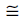ΔPQR.
Justify the statement by writing the congruence R

criteria applicable in this situation.

(b) In fig. ∠BAC = ∠QRP.
Justify that ΔABCΔRQP.

3. In ΔABC, AB = AC. OB and OC are bisectors of ∠B and ∠C respectively. Show that OB = OC.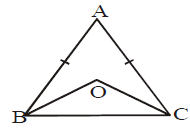4. In fig, ∠1 > ∠2. Show that AB > AC.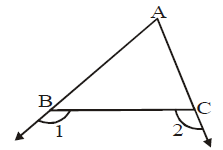5. In ΔABC, we have, ∠A > ∠B > ∠C, then determine the shortest and the longest side of the triangle.
6. If ΔABCΔPQR, ∠B = 40° and ∠C = 95°, find ∠P.
7. In ΔABC, AB = BC = 5cm and ∠A = 55°, find ∠B.
8. State the angle-angle-side congruence criteria for triangles.
9. In fig, AB = AC and ∠ACD = 115°. Find ∠A.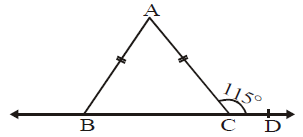10. In ΔABC, BC = AC and ∠B = 64°, find ∠C.
11. In ΔPQR, ∠P = 50° and ∠R = 70°. Name : (i) the shortest side (ii) the longest side of the triangle.

1. In the given fig, the line segments AB and CD intersect at a point M in such a way that AM = MD and CM = MB. Prove that, AC = BD but AC may not be parallel to BD.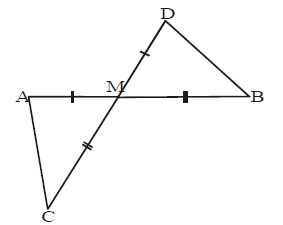2. In the given fig. AY ⊥ ZY and BY ⊥ XY such that AY = ZY and BY = XY. Prove that AB = ZX.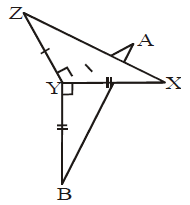3. If the bisector of the exterior vertical angle of a triangle is parallel to the base, show that the triangle is isosceles.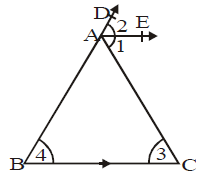4. In each of the following figures, find the value of x:

(i)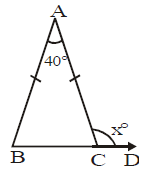(ii)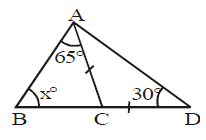5. In each of the following figures, find the value of x:

(i)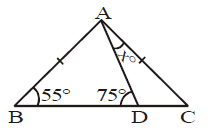(ii)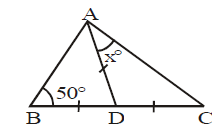6. In the given fig, BDCE; AC = BC, ∠ABD = 20° and ∠ECF = 70°. Find ∠GAC.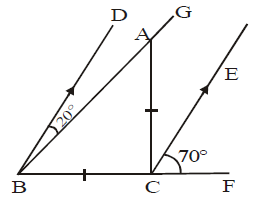7. In the given figure, ABCD and CA = CE. Find the values of x, y and z.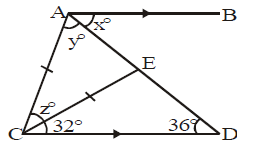8. In the given figure, AB = AD; CB = CD; ∠A = 42° and ∠C = 108°, find ∠ABC.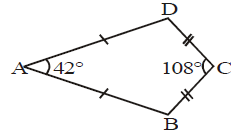9. In the given figure, side BA of ΔABC has been produced to D such that CD = CA and side CB has been produced to E. If ∠BAC = 106° and ∠ABE = 128°, find ∠BCD.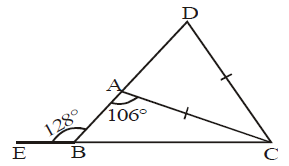10. In the given figure, AB = BC and AC = CD. Show that ∠BAD : ∠ADB = 3 : 1.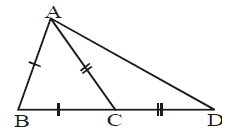11. In the given figure, AD is the internal bisector of ∠A and CEDA. If CE meets BA produced at E, prove that ΔCAE is isosceles.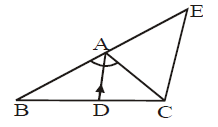12. In the given figure, AD bisects ∠A. Arrange AB, BD and DC in ascending order.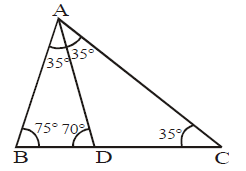13. In the given fig. AB = AC. Prove that : AF > AE.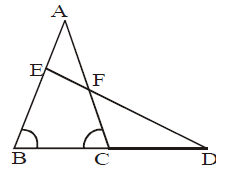14. In the given figure, side AB of ΔABC is produced to D such that BD = BC.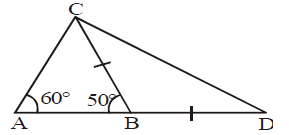If ∠A = 60° and ∠B = 50°, prove that :

15. In the given figure, AD bisects ∠A. If ∠B = 60°, ∠C = 40°, then arrange AB, BD and DC in ascending order of their lengths.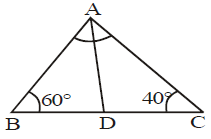(B) LONG ANSWER TYPE QUESTIONS :
1. In the given fig, ABCD is a square and ΔPAB is an equilateral triangle.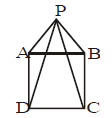(i) Prove that ΔAPD  ΔBPC.

(ii) Show that ∠DPC = 15°.

2. In the given fig, in ΔABC, ∠B = 90°. if ABPQ and ACRS are squares, prove that :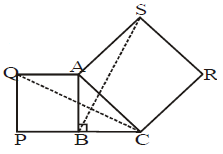(i) ΔACQ  ΔABS.
(ii) CQ = BS.

3. Squares ABPQ and ADRS are drawn on the sides AB and AD of a parallelogram ABCD. Prove that :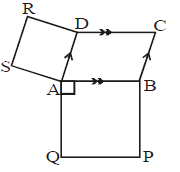(i) ∠SAQ = ∠ABC
(ii) SQ = AC.

4. In the given fig, ABCD is a square and P, Q, R are points on AB, BC and CD respectively such that AP = BQ= CR and ∠PQR = 90°. Prove that : (i) PB = QC, (ii) PQ = QR, (iii) ∠QPR = 45°.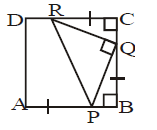5. In the given fig, ABCD is a square, EFBD and R is the mid-point of EF. Prove that :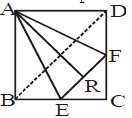(i) BE = DF

(iii) If AR is produced, it will pass through C.

6. In a ΔABC, AB = AC and BC is produced to D. From D, DE is drawn perpendicular to BA produced and DF is drawn perpendicular to AC produced. Prove that BD bisects ∠EDF.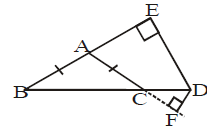7. Prove that the perimeter of a triangle is greater than the sum of its three medians.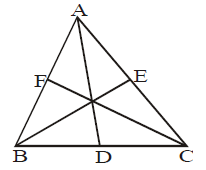8. In the adjoining figure, prove that :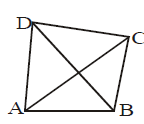(i) AB + BC + CD > DA
(ii) AB + BC + CD + DA > 2AC

(iii) AB + BC + CD + DA > 2BD
(iv) AB + BC + CD + DA > AC + BD

9. In the adjoining figure, O is the centre of a circle, XY is a diameter and XZ is a chord. Prove that XY > XZ.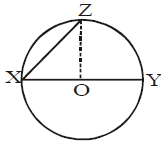10. In the given figure, AD = AB and AE bisects ∠A. Prove that :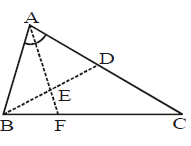(i) BE = ED

(ii) ∠ABD > ∠BCA.

VERY SHORT ANSWER TYPE QUESTIONS :
1. (a), (c)

2. (a) SAS congruence criteria

5. Shortest side is AB and the longest side is BC.
6. 45°
7. 70°
9. 50°
10. 52°
11. (i) QR,    (ii) PQ

4. (i) 110, (ii) 55
5. (i) 22, (ii) 40

6. 130°
7. x = 36, y = 68, z = 44
8. 105°
9. 54°
12. BD < AB < DC
15. BD = DC < AB

FILL IN THE BLANKS :

(a) Equal
(b) Equal
(c) Equal to
(d) AC
(e) EFD
(f) 20°
(g) RQ
(h) Included
(i) Three
(j) QRP
(k) FDE
(l) FDE
(m) Greater
(n) Smaller
(o) Perpendicular
(p) Less
(q) Greater
(r) Less
(s) Largest
(t) Greater

,

,

,

,

,

,

,

,

,

,

,

,

,

,

,

,

,

,

,

,

,

,

,

,

;